Courses

# Surveying (Part - 5) Civil Engineering (CE) Notes | EduRev

## Civil Engineering (CE) : Surveying (Part - 5) Civil Engineering (CE) Notes | EduRev

The document Surveying (Part - 5) Civil Engineering (CE) Notes | EduRev is a part of the Civil Engineering (CE) Course Civil Engineering SSC JE (Technical).
All you need of Civil Engineering (CE) at this link: Civil Engineering (CE)

Surveying (Part 5)

Bearing: The horizontal angle between and the reference meridian the survey line measure: in a clockwise direction, is called bearing.
The meridians of reference directions employed in surveying may one of the following.
1. True meridian
2. Magnetic meridian
3. Grid meridian
4. Arbitrary direction

True Meridian : The true or geographical meridian for a point on earth surface is the line which a plane passing through that point and true north and south pole intersects with the surface of the earth.
Magnetic Meridian : The magnetic meridian for a point on earth surface is the line in which a plane passing through that point and the magnetic north and south intersects with that surface of the earth.
True north : The surface of earth intersected by its axis yield two points namely None Geographical pole and South Geographical pole. This geographical north is called true north.
Magnetic north : Magnetic North and South line is determined as indicated by a free supported and properly balanced magnetic needle.

The maps prepared by the national survey department of any country, are based on true meridian.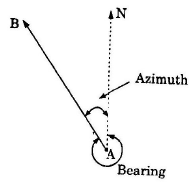True bearing: The horizontal angle between the true meridian and the survey line measured in a clockwise direction, is called true bearing of the line. It is also sometimes called azimuth.
Azimuth : The smaller angle which a survey Bearing line makes with the true meridian, is called “azimuth”.
If given true bearing of the line is 275°41then find its azimuth.
Solution. Azimuth of line = 360° — 375° 151 = 84° 15 If given true bearing of the line = 30°451 which is less than 180°, then find its azimuth. Solution. Azimuth of the line = True bearing of the line = 30°45
Magnetic bearing : The horizontal angle which a line makes with the magnetic meridian, is called magnetic bearing. It is not constant at a point but varies with laps of time.

Designation of Bearings: Bearing of survey lines are designated in the following system:
(i) The whole circle bearing system (W.C.B.)
(ii) The quadrental bearing system (Q.B.)

(1) The whole circle bearing system (W.C.B.) : The whole circle bearing system is also sometimes known as acimuthal system. In this system bearing of a line is measured from the true north or magnetic north in clockwise direction. The value of bearing may vary from 0° to 360°.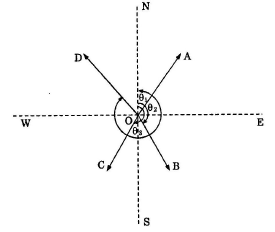W.C.B. of line OA, OB, OC and OD are q1,q2, qand q4 respectively.
(2) The Quadrantal bearings system (Q.B.) : In quadrantal bearing system, bearing of survey lines are measured eastward or westward from North and South whichever is nearest.
Bearing designated by Q.B. system, are sometimes called. Reduced Bearings (R.B.) The numberical value of a quadrantal bearings may vary from 0º to 90º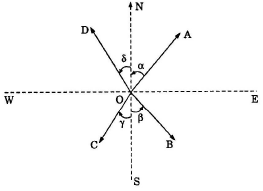Q.B. of a line OA, OB, OC and OD are designated as NaºE, SbºE, SgºW and NdºS, respectively.

Conversion of bearing from one system to the other : Suppose W.C.B of any line is 175º Q.B. of the line = 180 – 175º = 5º

hence the line is lying in SE Quadrant. It is also nearer to the south direction.
Hence the Q.B. of the line is designated as 55º E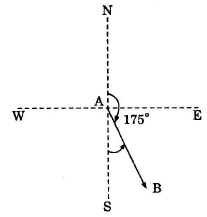Convertin W.C. bearing into Reduced bearing or Q.B.

 Case W.C.B. between Rule of Q.B. or R.B. Quadrant I 0º and 90º W.C.B N.E. II 90º and 180º 180 – W.C.B. S.E III 180º and 270º W.C.B. – 180 S.W. IV 270º and 360º 360º – W.C.B N.W.

Note: When a line lies exactly either along North. south,, East west, the W.C.B. of the line is converted in the quadrantal system as follows.

If W.C.B. of a line = 0º, then Q.B. of the line is N W.C.B. of a line = 90º, then Q.B. of the line is E 90º W.C.B. of a line = 180º, then Q.B. of the line is S W.C.B. of a line = 270º, then Q.B. of the line is W 90º.

Fore and Back Bearings : Every line may be defined by two bearings. Both the bearing are expressed in W.C.B. system and differ each other by 180º.
The bearing of a line in the direction of the program of survey is called Fore or Forward Bearing (F.B) While the bearing in the opposite direction of the progress of survey, is known as Reverse or Back Bearing (B.B)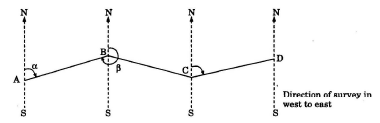In the above figure the bearing of line AB in the direction from A to B is a fore bearing

(a) whereas the bearing of the line AB in the direction from B to A a back bearing

(b).
Þ Back bearing = Fore bearing ± 180º, using + ve sign if the fore bearing is less than 180º and – ve sign if it is more than 180º –and – ve sign if it is mote than 180º in W.C.B. System 

• To convert the fore bearing of a line into its back bearing is Q.B. system, replace N by S, S by N, E by W and W by E, without changing the numberical value of the bearing Let the fore bearing of a line AB = N aº E \ Back bearing of a line AB = S aº WW Calculation of included angles from bearings:

(I) Given W.C.B. of lines included angle BAC = ÐNAC -ÐNAB = b – a Included angle BAC = Bearing of AC – Bearing of AB = different in bearing of AC and AB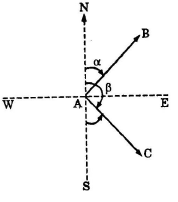(II) Given Q.B. of lines

(a)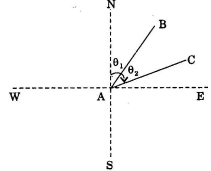Included angle = q2 – q1

(b)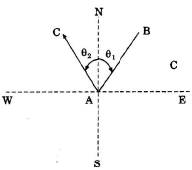Included angle =q2 + q1

(c)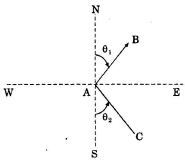Included angle = 180 – (q2 – q1)

(d)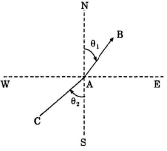the included angle = 180 + q1 – q2 = 180 – (q2 – q1)

Note:

(i) In a closed traverse run in anticlockwise direction, the observed included angles are interior angles.
(ii) In a closed traverse run in clockwise direction, the observing included angles, are exterior 

• The included angle of the lines is always less than 180°. If we calculate included angle and it is found greater than 180° than it substract from 360°.

LOCAL ATTRACTION :
North end of a freely suspended magnetic needle always points to the magnetic north, if it not influenced by any other external forces except the earth magnetic field. It is a common  experience that the magnetic needle gets deflected from its normal position, if placed near magnetic rocks, iron ores, cable carrying current or iron electric poles. Such a disturbance force is known as local attraction.

Detection of Local attraction : The presence of local attraction at any station may : detected by observing the fore and back bearing of the line. If the difference between fore an: back bearing is 180°, both end stations are free from local attention and if not, the discrepan: may be due to :

(i) an error in observation of either fore or back bearing or both.

(ii) presence of local attraction at either station.

(iii) presence of local attraction at both the stations.
The correction may be made according to the following methods

(i) By calculating the included angles at the affected stations.
(ii) By calculating the local attraction of each station, and poles, applying the require: corrections, starting from the unaffected bearing.

Sum of the exterior angles of the polygon = (2n 4)
Error = Observed back bearing = Calculated back braring.

Magnetic Declination : The horizontal angle between true north and magnetic north at a place at the time of observed is called magnetic declination.

Offer running on EduRev: Apply code STAYHOME200 to get INR 200 off on our premium plan EduRev Infinity!

## Civil Engineering SSC JE (Technical)

109 docs|50 tests

,

,

,

,

,

,

,

,

,

,

,

,

,

,

,

,

,

,

,

,

,

;